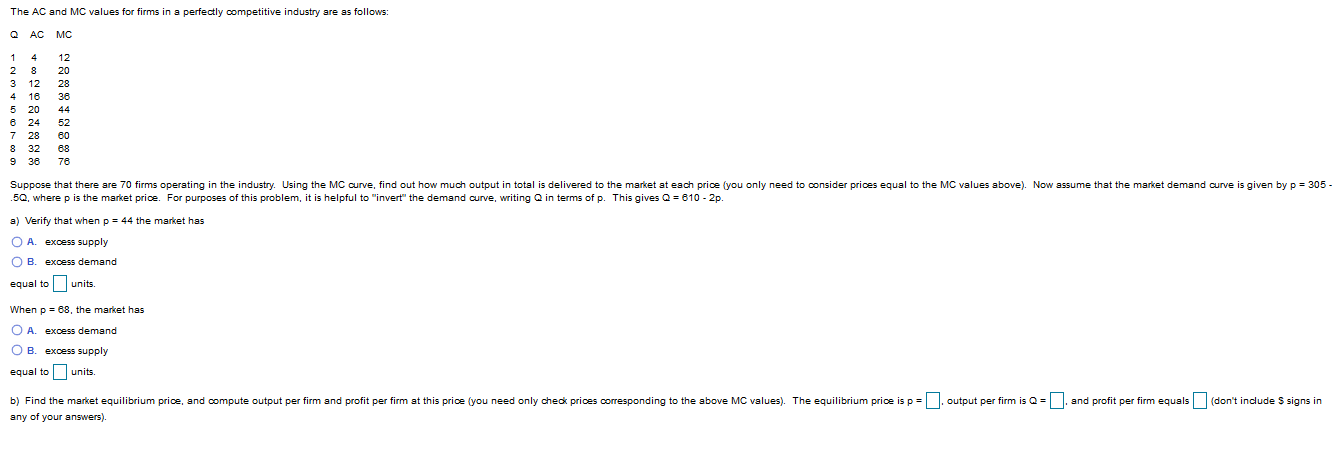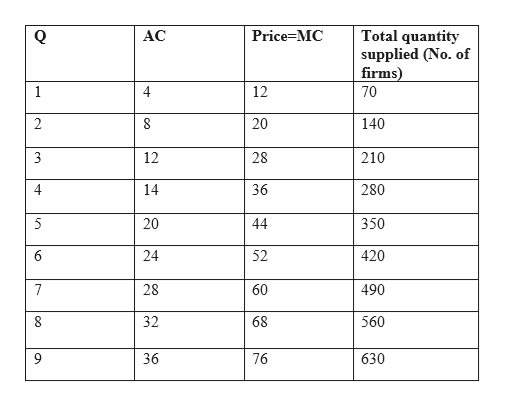# The AC and MC values for firms in a perfectly competitive industry are as follows:QAC MC1122 8203 124 16365 208 24527 28608 32683676Suppose that there are 70 firms operating in the industry. Using the MC curve, find out how much output in total is delivered to the market at each price (you only need to consider prices equal to the MC values above). Now assume that the market demand curve is given by p 305.5Q, where p is the market price. For purposes of this problem, it is helpful to "invert" the demand curve, writing Q in terms of p. This gives Q=610 2pa) Verify that when p 44 the market hasO A.excess supplyO B.excess demandequal to units.When p 68, the market hasO A.excess demandO B. excess supplyequal to units.and profit per firm equals(don't include S signs inb) Find the market equilibrium price, and compute output per firm and profit per firm at this price (you need only check prices corresponding to the above MC values. The equilibrium price is poutput per firm is Q=any of your answers).

Question
26 viewshelp_outlineImage TranscriptioncloseThe AC and MC values for firms in a perfectly competitive industry are as follows: Q AC MC 1 12 2 8 20 3 12 4 16 36 5 20 8 24 52 7 28 60 8 32 68 36 76 Suppose that there are 70 firms operating in the industry. Using the MC curve, find out how much output in total is delivered to the market at each price (you only need to consider prices equal to the MC values above). Now assume that the market demand curve is given by p 305 .5Q, where p is the market price. For purposes of this problem, it is helpful to "invert" the demand curve, writing Q in terms of p. This gives Q=610 2p a) Verify that when p 44 the market has O A. excess supply O B. excess demand equal to units. When p 68, the market has O A. excess demand O B. excess supply equal to units. and profit per firm equals(don't include S signs in b) Find the market equilibrium price, and compute output per firm and profit per firm at this price (you need only check prices corresponding to the above MC values. The equilibrium price is p output per firm is Q= any of your answers). fullscreen
check_circle

Step 1

In perfect competition, the firm produces at where price (or MR) equal to the marginal cost. It is given that the total number of firms are 70; so that the total quantity supplied can be find out as follows:help_outlineImage TranscriptioncloseTotal quantity supplied (No. of firms) АC Price MC 1 4 12 70 2 8 20 140 3 12 28 210 4 14 36 280 5 20 44 350 24 52 420 28 60 490 32 68 560 630 36 76 co CO O fullscreen
Step 2

a) When price is 44, the total quantity supplied is equal to 350, corresponding quantity demanded can be calculated by substituting the value of P in demand function as follows:

Step 3

Thus the quantity demanded is 522, which is greater than the quantity supplied. That means at price 44, market has excess demand equal to 172 units (522-350).

When price is 68, t...

### Want to see the full answer?

See Solution

#### Want to see this answer and more?

Solutions are written by subject experts who are available 24/7. Questions are typically answered within 1 hour.*

See Solution
*Response times may vary by subject and question.
Tagged in